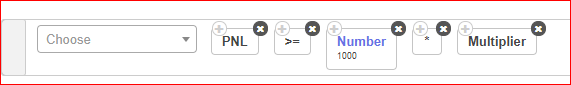# "PNL / Target / Stoploss" related keywords: Different Examples of its Uses

This thread will contain all details about the usage of “PNL” keyword, “PNL Underlying” Keyword, “Max Profit” keyword etc and its different examples:

1 Like

PNL Keyword Calculates PNL of the “Entire” Strategy, so even if you use the PNL in Set, PNL of entire strategy will be calculated and accordingly the strategy condition will be executed.

Note that same applies for “PNL Underlying” keyword, however, PNL Underlying keyword will calculate PNL only of the particular stock mentioned in the list of PNL Underlying i.e. will calculate the profit for each stock separately in the list. It will not calculate PNL of all stocks combined in the PNL Underlying list.

The “PNL Underlying” is to be used in set exit, whereas PNL keyword is to be used only in Universal Exit.

More on PNL Underlying Keyword:

“PNL Underlying” Keyword for List of Stocks on Tradetron

1. Max Profit Keyword:

Kindly watch this video:

Example 1:

Also, please make sure to use brackets properly:
Example 2:

Example 3:
In following Example, when Max profit reaches 500, the condition will be activated and when the profit goes below Max profit - the defined number, it will exit the position
Eg: 500 - 100 = 400.

Example 4:
Below is an example of:
When Max profit reaches Rs. 150, I want to trail it by 36 Rs.:

Example 5:
Another use of Max Profit Keyword Can be as below:

So as per the log generated for this condition:
Max_pnl ( ‘5318525’, ‘25’ ) : 956.2501525878906
PNL ( ‘5318525’, ‘25’ ) : -182.49984741210938

Whenever the PNL goes below the Highest PNL (Max PNL) reached for the counter by 1100 Rs, the strategy will automatically exit. As seen above, the max profit reached was 956.25, then after that when if fell by 1100 points, from max profit to -182.49, the strategy exited.

1 Like

What is the use of “Multiplier” Keyword:

In deployed page, you can customise the multiplier according to your choice, what it does is , multiplies the qty by that amount when executing a trade. So when you add this condition in PNL it multiplies the amount by the same multiplier you deployed with.For eg:

Standard pnl condition is 1500
When used multiplier in deployed as 2x
Pnl condition is 1500 x 2 = 3000Question: Leg Exit based on PNL:

Below is an example of calculating PNL for a particular traded leg:

In the below image:

1. (NIFTY 50, 1,1,1) refers to the already traded instrument in Set No.1, Condition No. 1 and Leg No. 1: For which the PNL needs to be greater than or equal to Rs. 2000

Please make sure to use brackets properly.

Question: Set Exit Based on Target or Stoploss of Rs. 500.

Solution:
This is based on assumption that positions are Short Call as (NIFTY BANK, 1,1,1) and Shot Put as (NIFTY BANK, 1,1,2).

Question: For a Call Option shorted or sold in Set 1, Condition 1, Leg 1 as (NIFTY BANK, 1,1,1), I want to exit the short position if the price moves up between 20% and 60% only:

Solution:

In Short Straddle / Strangle Position: How to move SL to Cost of One leg after the other leg has hit Stop loss:

Now, the First Repair will be constructed as follows:
Here we check 2 Conditions:

1. Independent stop loss for Leg 1, i.e. Call Leg is set at 10 points higher than its Entry price.
2. In the 2nd part below: We are first checking if the Put Leg SL (which is in 2nd Repair Once of set 1) has been traded, i.e. SL is hit with ‘Traded Instrument’ keyword. Also, we are checking that the Net Quantity of the Put leg is now 0, means it is squared off. Hence, we will move the SL of the Call Leg to Cost by using the formula: LTP (Traded Instrument Name… 1,1,1) > Traded Instrument (Entry, Price, 1,1,1).

Similarly, the Second Repair will be constructed as follows:
Here we check 2 Conditions:
3. Independent stop loss for Leg 2, i.e. Put Leg, is set at 10 points higher than its Entry price.
4. In the 2nd part below: We are first checking if the Call Leg SL (which is in 1st Repair Once of set 1) has been traded, i.e. SL is hit with ‘Traded Instrument’ keyword. Also, we are checking that the Net Quantity of the Call leg is now 0, means it is squared off. Hence, we will move the SL of the Put Leg to Cost by using the formula: LTP (Traded Instrument Name… 1,1,2) > Traded Instrument (Entry, Price, 1,1,2).

Below is an example of the short leg, for which the stoploss is being placed at 50% of the shorted price, for the leg number 2 of set 1.

Below is an example of the short leg, for which the stoploss is being placed at above 20 points of the shorted price, for the leg number 1 of set 1.

How to use “Traded Instrument (PNL) Keyword” to calculate pnl of a particular leg. Please watch the below video